9.4. SIMULATION OF OIL EXTRACTION

9.3. NUMERICAL INTEGRATION

In this section we extend the problem of numerical approximation to definite integrals on heterogeneous networks of computers. First, we briefly introduce numerical integration, following the excellent book Computer Methods for Mathematical Computations by Forsythe, Malcolm, and Moler (1977). Then we give a parallel algorithm of numerical integration on heterogeneous networks as well as its mpC implementation.

To avoid confusion with numerical integration of ordinary differential equations, the term quadrature is commonly used for numerical approximation of definite integrals. Let [a,b] be a finite interval on the x axis that is partitioned into n subintervals called panels, [xi, xi1], i = 0,. . ., n - 1. Assume x0 = a, xn = b, and x0 < x1 <...< xn. Let hi = xi1 - xi be the panel widths.

Let f(x) be a function defined on [a,b]. Suppose that an approximation is desired to the definite integral

numerical integration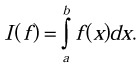Clearly, I(f) can be expressed as a sum of integrals over the panels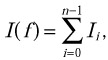whereA quadrature rule is a simple formula for approximating the individual Ii. A composite quadrature rule is a formula giving an approximation to I(f) as a sum of the quadrature rule approximations to the individual Ii. Two of the simplest quadrature rules are the rectangle rule and the trapezoid rule.

9.3.1. Basic Quadrature Rules

The rectangle rule uses function values in the midpoints of the panels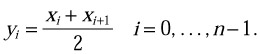It approximates each Ii by the area of the rectangle with base hi and height f(yi). This gives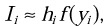and hence we have the composite rectangle rule: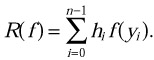The trapezoid rule uses function values at the end points of the panels. It approximates Ii by the area of the trapezoid with base hi and height that varies linearly from f(xi) on the left to f(xi1) on the right. This leads to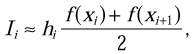and hence we have the composite trapezoid rule:It is easily shown that if f(x) is continuous (or even merely Riemann integrable) on [a,b] and h = maxi hi, then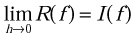and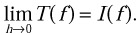That is, both rules converge as the lengths of the subintervals decrease.

The rectangle rule is based on piecewise constant interpolation, while the trapezoid rule is based on piecewise linear interpolation. One might therefore expect the trapezoid rule to be more accurate that the rectangle rule. But this natural expectation does not always prove true.

Indeed, assume that f has five continuous derivatives and that the values of these derivatives are not too large. Consider a panel [xi, xi1]. The Taylor series expansion of f(x) about yi, the center of this panel, is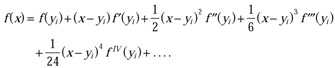These assumptions about f imply that the remainder term denoted by ellipses (...) is less significant than the terms explicitly shown.

To integrate this series over [xi, xi1], observe that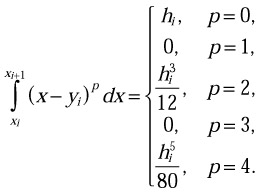Note that the odd powers integrate to zero. Consequently

numerical integration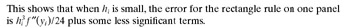Returning to the Taylor series and substituting x = xi and x = xi1, we obtain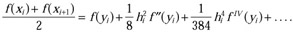Combining this with the expansion for the integral givesThis shows that when hi is small, the error for the trapezoid rule on one panel is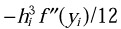plus some less significant terms.

The total error for either rule is the sum of the errors on the individual panels. Let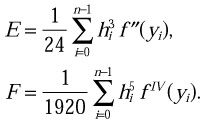Then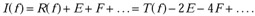If the hi are sufficiently small, then hi5 << hi3; so if fIV is not too badly behaved, then F << E.

The first conclusion from these results is that for many functions f(x), the rectangle rule is about twice as accurate as the trapezoid rule. The second conclusion is that the difference between the values obtained by the rectangle and trapezoid rules can be used to estimate the error in either one of them. The third conclusion is that, for functions f(x) having continuous, bounded second derivatives, doubling the number of panels by bisecting them in either the rectangle or trapezoid rule can be expected to roughly quadruple the accuracy. The difference in the results of either of these rules before and after doubling the number of panels can be used to estimate the error or improve the computed result.

The technique of repeatedly doubling the number of panels and estimating the error can be programmed to produce a method that automatically determines the panels so that the approximate integral is computed to within some prescribed error tolerance. Combining the rectangle and trapezoid rules in the proper way can produce a new rule, for which the error formula no longer contains E. Indeed, the error in the combined quadrature rule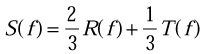is given by the formulaThis rule is called the composite Simpson’s rule. Notice that even though S(f) is based on second-degree interpolation, the error term involves the fourth derivative, and hence Simpson’s rule is exact for cubic functions. In other words, like the rectangle rule, Simpson’s rule obtains an “extra” order of accuracy.

If the length of each panel is halved, then each hi5 term in the error formula is decreased by a factor of 1/32. The total number of terms is increased by a factor of 2, so the overall error is decreased by a factor close to 1/16. This fact is important for programs that automatically choose the number and size of the panels.

An adaptive quadrature routine is a numerical quadrature algorithm that uses one or two basic quadrature rules and automatically determines the subinterval sizes so that the computed result meets some prescribed accuracy requirement. Different mesh sizes may be used in different parts of the interval. Relatively large meshes are used where the integrand is smooth, and slowly varying and relatively small meshes are used in regions where the integration becomes difficult. This way an attempt is made to provide a result with the prescribed accuracy at as small a cost in computer time as possible.

An adaptive quadrature routine normally requires that the user specify a finite interval [a,b], provide a subroutine that computes f(x) for any x in the interval, and choose an error tolerance e. The routine attempts to compute a quantity Q so that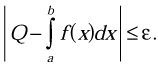The routine may decide that the prescribed accuracy is not attainable, do the best it can, and return an estimate of the accuracy actually achieved.

In assessing the efficiency of any quadrature routine, it is generally assumed that the major portion of the computation cost lies in evaluation of the integrand f(x). So, if two subroutines are given the same function to integrate and both produce answers of about the same accuracy, the subroutine that requires the smaller number of function evaluations is regarded as the more efficient in that particular problem.

It is always possible to invent integrands f(x) that “fool” the routine into producing a completely wrong result. However, for good adaptive routines the class of such examples has been made as small as possible without unduly complicating the logic of the routine or making it inefficient in handling reasonable problems.

During the computation the interval [a, b] is broken down into subintervals [xi, xi1]. Each subinterval is obtained by bisecting a subinterval obtained earlier in the computation. The actual number of subintervals, as well as their locations and lengths, depends on the integrand f(x) and the accuracy requirement e. The numbering is arranged so that x0 = a, and the routine determines an n so that xn = b. We let hi = xi1 - xi be the subinterval width.

A typical scheme applies two different quadrature rules to each subinterval. Let us denote the results by Pi and Qi. Both Pi and Qi are approximations to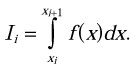The basic idea of adaptive quadrature is to compare the two approximations Pi and Qi and thereby obtain an estimate of their accuracy. If the accuracy is acceptable, one of them is taken as the value of the integral over the subinterval. If the accuracy is not acceptable, the subinterval is divided into two parts and the process repeated on the smaller subintervals.

To reduce the total number of function evaluations, it is usually arranged that the two rules producing Pi and Qi require integrand values at some of the same points. Consequently processing a new subinterval normally requires about the same number of new function evaluations.

For simplicity we assume that Qi is obtained simply by applying the rule for Pi twice, once to each half of the interval. Let us also assume that the rule for Pi gives the exact answer if the integrand is a polynomial of degree p - 1, or equivalently, if the p-th derivative f(p)(x) is identically zero. By expanding the integrand in a Taylor series about the midpoint of the subinterval, we can then show that there is a constant c so that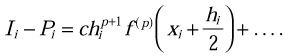The exponent of hi is p + 1 rather than p because the subinterval is of length hi. From the assumption that Qi is the sum of two P’s from two subintervals of length hi/2, it follows that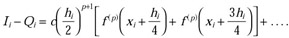Since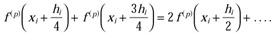and the two errors are related by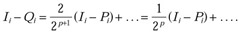This indicates that bisecting the subinterval decreases the error by a factor of about 2p. Solving for the unknown Ii and rearranging terms, we obtain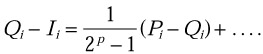In other words, the error in the more accurate approximation Qi is about 1/2p - 1 times the difference between the two approximations.

The basic task of a typical routine is to bisect each subinterval until the following inequality is satisfied:where e is the user-supplied accuracy tolerance. If the entire interval [a, b] can be covered by n subintervals, on which this is valid, then the routine would returnCombining the above and ignoring the higher-order terms, we find that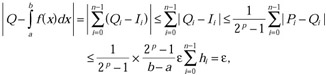which is the desired goal.

This analysis requires the assumptions that f(p)(x) be continuous and that the error be proportional to hip1 f(p)(x). Even when these conditions are not exactly satisfied, the routine may perform well, and the final result may be within the desired tolerance. However, the detailed behavior will be different.

For simplicity we have also assumed that the routine is using an absolute error criterion, that is,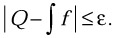In many situations the preferred error tolerance is the relative kind, which is independent of the scale factors in f. The pure relative criterion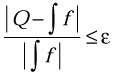is complicated by several factors. The denominator f might be zero. Even if the denominator is merely close to zero because the positive values of f at one part of the interval nearly cancel the negative values of f at another part, the criterion may be impossible to satisfy in practice. Furthermore a good approximation to the value of the denominator is not available until the end of the computation

Some routines use a criterion involving |f|,The denominator cannot be zero unless f is identically zero and does not suffer the cancellation difficulties associated with oscillatory integrands. However, this route does involve more computation, although no more function evaluations, and does require a more elaborate explanation.

One of the most popular adaptive quadrature routines is a routine called quanc8. The user of the routine must supply a function fun for the integrand f(x); the lower and upper limits of integration, a and b; and the absolute and relative error tolerances, abserr and relerr. The routine returns the approximation of the integral; an estimate of the absolute error, errest; the number of function evaluations required, nofun; and a reliability indicator, flag. If flag is zero, then the approximation likely has the desired accuracy. If flag is large, then some unacceptable integrand has been encountered.

The name quanc8 is derived from quadrature, adaptive, Newton-Cotes’s 8-panel. The Newton-Cotes formulas are a family of quadrature rules that are obtained by integrating interpolating polynomials over equally spaced evaluation points. The rectangle, trapezoid, and Simpson’s rules are obtained by integrating zeroth-, first-, and second-degree polynomials, and they constitute the first three members of the family. The eighth-panel formula is obtained by integrating an eighth-degree polynomial, but just as by the rectangle and Simpson’s rules, an “extra” degree is acquired, so the formula gives the exact result for polynomials of degree 9.

For integration over the interval [0, 1] the rule is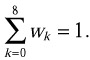Rational expressions for the weights wk (multiplied by 8) are included in the routine itself. Since the rule is exact for f(x) 1, the weights satisfyHowever, some of the weights are negative, and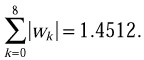This factor is important in error analysis. For example, suppose that function f(x) 1 is contaminated by some error factor so that f(x) = 0.99 at x = 2/8, 4/8, 6/8, and f(x) = 1.01 at all other nodes. The eight-panel rule would give 1.014512 as the value of the integral. The error in the function values is slightly magnified in the result. The magnification is inconsequential in this rule, but for the Newton-Cotes formulas involving higher polynomials, this sort of error is unacceptable.

A rule with eight panels has been chosen so that each panel width is the basic interval width, b - a, divided by a power of 2. Consequently there are usually no round-off errors in computing the nodes xi on a computer.

The eight-panel rule is used in quanc8 to obtain the quantity Pi. The quantity Qi is obtained by applying the same rule to the two halves of the interval, thereby using 16 panels. In the program, variables qprev and qnow are used for Pi and Qi. Variables qleft and qright are used for the results on the two halves of the interval.

Since the basic rule is exact for ninth-degree polynomials, the error in Qi will be about 1/(210 - 1) = 1/1023 times the error in Pi whenever the integrand is smooth and higher-order terms can be ignored. Thus the results for a particular subinterval are acceptable ifIn quanc8 this test is made by comparing two quantities

esterr = fabs(qnow-qprev)/1023.

and

tollerr = fmax(abserr, relerr*fabs(area))*(step/stone).

The ratio step/stone is equal to hi/(b - a), and area is an estimate of the integral over the entire interval, so e is the maximum of abserr and relerr*| f|. The subinterval is acceptable if esterr<=tolerr.

When the subinterval is accepted, qnow is added to result, and esterr is added to errest. Then the quantity (qnow-qprev)/1023 is added to variable cor11 because Qi + (Qi - Pi)/1023 is usually a more accurate estimate of Ii than simply Qi. The final value of corr11 is added to result just before its value is returned.

Each time a subinterval is bisected, the nodes and function values for the right half are saved for later use. Limit levmax=30 is set on the level of bisection. When this maximum is reached, the subinterval is accepted even if the estimated error is too large, but a count of the number of such subintervals is kept and returned as the integer part of flag. The length of each of these subintervals is quite small, (b - a)/230, so for most integrands a few of subintervals can be included in the final result without affecting accuracy much.

However, if the integrand has discontinuities or unbounded derivatives, or if it is contaminated by round-off error, the bisection level maximum will be frequently reached. Consequently another limit, nomax, is set on the number of function evaluations. When it appears that this limit may be exceeded, levmax is reduced to levout so that the remainder of the interval can be processed with fewer than nomax function evaluations. Furthermore the point x0 where the trouble is encountered is noted and the quantity (b-x0)/(b-a) is returned as the fractional part of flag. This is the portion of the interval that remains to be processed with the lower bisection limit.

As based on piecewise polynomial approximation, quanc8 is not designed to handle certain kinds of integrals. Roughly these are integrals of functions f(x) for which some derivative f(k)(x) with k 10 is unbounded or fails to exist.

The C code of quanc8 is as follows:

#define fmax(a,b) ((a)>(b)?(a):(b)) // Quanc8 estimates the integral of fun(x) from a to b to a user // provided tolerance (a relative error tolerance, relerr, and/or // an absolute error tolerance, abserr). Quanc8 is an automatic // adaptive routine based on the 8-panel Newton-Cotes rule. // It returns an approximation to the integral hopefully // satisfying the least stringent of the two error tolerances. // An estimate of the magnitude of the actual error is returned // in variable pointed by errest. The number of function values // used in calculation of the result is returned in variable // pointed by nofun. A reliability indicator is returned in // variable pointed by flag. If it is zero, then the result // probably satisfies the error tolerance. If it is x.y, then x // is the number of intervals that have not converged and 0.y // is the fraction of the interval left to do when the limit on // nofun was approached. double quanc8(double (*fun)(double), double a, double b,    double abserr, double relerr, double *errest,    int *nofun, double *flag) {    static double w[] = {3956.0/14175.0, 23552.0/14175.0,    -3712.0/14175.0, 41984.0/14175.0,    -18160.0/14175.0};    double area=0., result=0., stone, step, cor11=0.0,     temp;    double qprev=0.0, qnow, qdiff, qleft, esterr, tollerr;    double qright, f, x, fsave, xsave;    int levmin=1, levmax=30, levout=6, nomax=5000, nofin,     lev=0,nim=1, i, j;    if(a==b)       return result;       // Initialization       nofin = nomax-8*(levmax-levout+(int)pow(2,        levout+1));       *flag=0;       *errest=0.0;       *nofun=0;       x = a;       x = b;       f = (*fun)(x);       stone = (b-a)/16.0;       x = (x+x)/2.0;       x = (x+x)/2.0;       x = (x+x)/2.0;       x = (x+x)/2.0;       x = (x+x)/2.0;       x = (x+x)/2.0;       x = (x+x)/2.0;       for(j=2; j<17; j+=2)          f[j] = (*fun)(x[j]);       *nofun = 9;       // Central calculation    m30:       for (j=1; j<16; j+=2)       {          x[j] = (x[j-1]+x[j+1])/2.0;          f[j] = (*fun)(x[j]);       }       *nofun += 8;       step = (x-x)/16.0;       qleft = (w*(f+f)+             w*(f+f)+             w*(f+f)+             w*(f+f)+             w*f             )*step;       qright[lev] = (w*(f+f)+             w*(f+f)+             w*(f+f)+             w*(f+f)+             w*f             )*step;       qnow = qleft+qright[lev];       qdiff = qnow-qprev;       area = area+qdiff;       // Interval convergence test       esterr = fabs(qdiff)/1023.0;       tollerr = fmax(abserr, relerr*fabs(area))*(step/stone);       if(lev<levmin)          goto m50;       if(lev>=levmax)          goto m62;       if(*nofun>nofin)          goto m60;       if(esterr<=tollerr)          goto m70;       // Locate next interval and save right-hand elements for future       // use    m50:       nim *= 2;       for(i=0; i<8; i++)       {          fsave[lev][i] = f[i+9];          xsave[lev][i] = x[i+9];       }       lev++;       // Assemble left-hand elements for immediate use       qprev = qleft;       for(i=-1; i>-9; i--)       {          f[2*i+18] = f[i+9];          x[2*i+18] = x[i+9];       }       goto m30;       // Trouble: number of function values is about to exceed limit.    m60:       nofin *= 2;       levmax = levout;       *flag += (b-x)/(b-a);       goto m70;       // Trouble: current level is levmax.    m62:       *flag += 1.0;       // Interval converged: add contributions into running sums.    m70:       result += qnow;       *errest += esterr;       cor11 += qdiff/1023.0;       // Locate next interval.    m72:       if(nim==2*(nim/2))          goto m75;       nim/=2;       lev--;       goto m72;    m75:       nim++;       if(lev<=0)          goto m80;       // Assemble elements required for the next interval.       qprev = qright[lev-1];       x = x;       f = f;       for(i=1; i<9; i++)       {          f[2*i] = fsave[lev-1][i-1];          x[2*i] = xsave[lev-1][i-1];       }       goto m30;       // Finalize and return: make sure that errest not less than       // round-off level.    m80:       result+=cor11;       if(!*errest)          return result;    m82:       temp = fabs(result)+*errest;       if(temp!=fabs(result))          return result;          *errest*=2.0;          goto m82; }

9.3.4. Parallel Adaptive Quadrature Routine for Heterogeneous Clusters

The best adaptive quadrature routines are designed to provide an approximation to definite integrals with the prescribed accuracy in the fastest possible computer time. The large portion of the computation cost lies in evaluation of the integrand f(x). Therefore, to achieve the best efficiency on serial computers, the programmer should minimize the total number of function evaluations.

If the evaluation of integrand f(x) is prohibitively expensive, the speedup may be achieved via parallel computing of the integrand values. To do this, we let tp(f) be the time of evaluation of f(x) at a single point on a processor p. We let tpq be the time of transfer of a single floating-point value from the processor p to a processor q. Say we have a cluster of heterogeneous processors, and the time of evaluation of f(x) on a single processor exceeds the time of transfer of a single floating-point value between any pair of the processors doubled,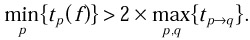We can write an algorithm of parallel evaluation of integrand f(x) at n different points on two processors p and q. We let n points be stored in the memory of processor p, and resulting integrand values be stored in the memory of this processor. Let sp(f) and sq(f) be the relative speed of processors p and q respectively, demonstrated on the evaluation of integrand f(x):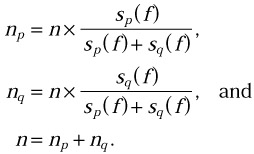• At the first step of the algorithm, processor p sends nq points to processor q.

• At the second step, processor p evaluates f(x) at np points, while processor q evaluates f(x) at the nq points received from processor p. Note that the number of function evaluations performed by each processor will be proportional to its relative speed, np/nq = sp(f)/sq(f). From the assumption that the relative speed is demonstrated on the evaluation of the integrand f(x), it follows that sp(f)/sq(f) = tq(f)/tp(f). Therefore np x tp(f) = nq x tq(f).

• Finally processor p receives nq integrand values from processor q and stores them in its memory.

The total time of serial evaluation of f(x) at n different points on the processor p will exceed the time of the parallel evaluation on a cluster of two processors, p and q: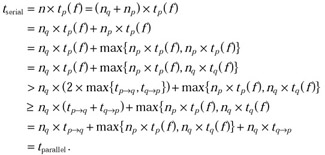The result can be easily generalized to a heterogeneous cluster consisting of an arbitrary number of processors.

There are different ways of designing parallel adaptive quadrature routines for a heterogeneous cluster of p processors. One simple way is to break up the interval [a, b] into p subintervals, with each subinterval assigned to a separate processor. The desired integral over the entire interval [a, b] is obtained as a sum of integrals over these subintervals. Each processor computes an estimate of the integral over its subinterval following the same serial adaptive quadrature routine. To balance the load of processors, one would make the length of the kth interval proportional to the relative speed of the kth processor.

This algorithm is quite simple and straightforward to implement. However, it balances the load of processors only if the same mesh size is used in all parts of the interval [a, b]. If different mesh sizes are used in different parts of this interval, the number of function evaluations performed by each processor will be no longer proportional to its relative speed, resulting in unbalanced loads processor.

This algorithm will not be efficient if integrand f(x) is smooth and slowly varying in some regions of interval [a, b] and has high peaks in other regions. If the same interval spacing required near the peaks to obtain the desired accuracy, is used throughout the entire interval [a, b], then many more function evaluations will be performed than is necessary (although the load of the processors will be balanced). If larger meshes are used in regions where the integrand is smooth and slowly varying, and smaller meshes are used in regions where the integration becomes difficult, then the balance between the loads of different processors will be broken. In both situations the actual computing time will be far longer from the optimal one. This is when we turn to a parallel adaptive quadrature routine, pquanc8, that tries to keep balance between loads of processors while minimizing the total number of function evaluations required to provide a result with the prescribed accuracy.

As it might be clear from its name, the pquonc8 routine is a parallel version of the quanc8 adaptive quadrature routine. The algorithm of parallel numerical integration on a cluster of p heterogeneous processors can be summarized as follows:

• The algorithm uses a master worker parallel programming paradigm. All computations, except for function evaluations, are performed serially by a host-processor.

• The host-processor keeps a list of subintervals of the entire interval [a, b], whose contributions to the integral are still to be estimated. Each subinterval [l, r] is represented by a data structure that consists of

• an array x to contain a set of points x0, x1, . . ., x16 so that x0 = l, x16 = r, x0 = 1 x16 = r. x0 < x1, < . . . < x16and xi1 - xi = (l - r)/16;

• an array f to contain a set of integrand values f(x) at these points; and

• a variable qprev to contain an estimate of the integral over the interval [l, r] obtained by using the eight-panel rule, that is, by using values f(x0), f(x2), f(x4), f(x6), f(x10), f(x12), f(x14), f(x16).

Initially the list consists of interval [a, b] and the host-processor computes the integrand values at even points x0, x2,..., x16 associated with the interval.

• At each iteration of the main loop of the algorithm, an estimate of the integral over each subinterval in the current list of subintervals is calculated. The estimate is obtained from the integrand values at all 17 points associated with the subinterval. If the subinterval is acceptable, its contribution is added to the result. If the subinterval is not acceptable, the interval is bisected into two new subintervals that are added to a list of subintervals to be processed at next iteration of the main loop. In other words, one step of routine quanc8 is applied to each subinterval in the current list. Note that at the beginning of the iteration all subintervals in the current list are of the same length.

• In order to estimate the integral over each subinterval in the current list, the host-processor needs integrand f(x) to be evaluated at odd points x1, x3,..., x15 of the subinterval. The estimation involves all processors of the cluster and is performed as follows:

• First the host-processor forms a set of all odd points associated with subintervals in the current list.

• Then it scatters the set across p processors of the cluster. The number of points received by each processor is proportional to the current estimation of its relative speed.

• Each processor estimates integrand f(x) at the points received form the host-processor. Besides making the estimation, the processor measures the time taken to do the estimation. Then it sends to the host-processor both the integrand values and the time taken to compute the values.

• The host-processor uses the computation time received from each processor to calculate their relative speeds demonstrated during this integrand evaluation. This fresh estimation of the relative speeds of processors will be used at the next iteration of the main loop. Note that this approach makes pquanc8 adaptive to possible changes in the speeds of the individual processors even during the execution of the program (i.e., due to some external applications).

The mpC implementation of routine pquanc8 takes the following form:

The preceding pquanc8 routine does not contain a serial code for calculating the number of points to be assigned to each processor and for processing the lists of subintervals. The code is kept in the files partition.h and interval.h respectively, and included in the mpC program with the include directives. Other two files included in the program are

• quanc8.h, which contains the C code of the quanc8 routine. The file is used in the program to compare the quanc8 execution time with the execution time of pquanc8.

• function.h, which contains the definition of a function fun for integrand f(x).

The full code of the mpC application can be found in Appendix C.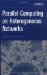Parallel Computing on Heterogeneous Networks (Wiley Series on Parallel and Distributed Computing)
ISBN: B000W7ZQBO
EAN: N/A
Year: 2005
Pages: 95